# NMath User's Guide

41.3 One Sample T-Test (.NET, C#, CSharp, VB, Visual Basic, F#)

Class determines whether a sample from a normal distribution with unknown standard deviation could have a given mean. For example, suppose we wish to determine whether the self-esteem of children from a particular school differ from average, given a known population value of 3.9 on the Rosenberg Self­-Esteem Scale. 113 children are tested, with a mean score of 4.0408 and a standard deviation of .6542.

As described Section 41.1, all hypothesis test classes provide two paths for constructing instances of that type: a parameter-based method and a data-based method. Thus, you can construct a OneSampleTTest object by explicitly specifying a sample mean (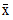), sample standard deviation (), sample size (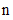), and population mean (), like so:

Code Example – C# t-test

```double xbar = 4.0408;
```
```double s = .6542;
```
```int n = 113;
```
```double mu0 = 3.9;
```
```var test = new OneSampleTTest( xbar, s, n, mu0 );
```

Code Example – VB t-test

```Dim XBar As Double = 4.0408
```
```Dim S As Double = 0.6542
```
```Dim N As Integer = 113
```
```Dim Mu0 As Double = 3.9
```
```Dim Test As New OneSampleTTest(XBar, S, N, Mu0)
```

Or by supplying a set of sample data, and the necessary population parameters. For instance, if the sample data is in column 3 of DataFrame df:

Code Example – C# t-test

```double mu0 = 3.9;
```
```var test = new OneSampleTTest( df, mu0 );
```

Code Example – VB t-test

```Dim Mu0 As Double = 3.9
```
```Dim Test As New OneSampleTTest(DF(3), Mu0)
```

In this case, the sample mean, standard deviation, and size are calculated from the given data.

In addition to the properties common to all hypothesis test objects (Section 41.1), a OneSampleTTest object provides the following read-only properties:

Xbar gets the sample mean.

S gets the sample standard deviation.

N gets the sample size.

Mu0 gets the population mean.

DegreesOfFreedom gets the degrees of freedom.

By default, a OneSampleTTest object performs a two-sided hypothesis test (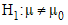) with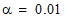. Non-default test parameters can be specified at the time of construction using constructor overloads, or after construction using the provided Alpha and Type properties, like so:

Code Example – C# t-test

```test.Alpha = 0.05;
```

Code Example – VB t-test

```Test.Alpha = 0.05
```

Once you've constructed and configured a OneSampleTTest object, you can access the various test results using the provided properties, as described in Section 41.1:

Code Example – C# t-test

```Console.WriteLine( "t-statistic = " + test.Statistic );
```
```Console.WriteLine( "deg of freedom = " + test.DegreesOfFreedom );
```
```Console.WriteLine( "p-value = " + test.P );
```
```Console.WriteLine( "reject the null hypothesis? " + test.Reject);
```

Code Example – VB t-test

```Console.WriteLine("t-statistic = " & Test.Statistic)
```
```Console.WriteLine("deg of freedom = " & Test.DegreesOfFreedom)
```
```Console.WriteLine("p-value = " & Test.P)
```
```Console.WriteLine("reject the null hypothesis? " & Test.Reject)
```

The output is:

```t-statistic = 2.28786996397591
```
```deg of freedom = 112
```
```p-value = 0.0240223660991041
```
```reject the null hypothesis? True
```

This indicates that we can reject the null hypotheses (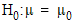). We can conclude that the children have self-esteem scores significantly different than average.

Finally, remember that the ToString() method returns a formatted string representation of the complete test results:

```One Sample t Test
```
```-----------------

```

```Sample mean = 4.0408
```
```Sample standard deviation = 0.6542
```
```Sample size = 113
```
```Population mean = 3.9
```
```Computed t statistic: 2.28786996397591, df = 112

```

```Hypothesis type: two-sided
```
```Null hypothesis: sample mean = population mean
```
```Alt hypothesis: sample mean != population mean
```
```P-value: 0.0240223660991041
```
```REJECT the null hypothesis for alpha = 0.05
```
```0.95 confidence interval: 3.91886249658971 4.16273750341029
```

Top

Top